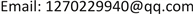﻿ 一致模糊极小集及其序同态 Uniformly Fuzzy Minimal Posets and Its Order Homomorphism

Operations Research and Fuzziology
Vol. 09  No. 01 ( 2019 ), Article ID: 28100 , 5 pages
10.12677/ORF.2019.91001

Uniformly Fuzzy Minimal Posets and Its Order Homomorphism

Lu Chen, Hui Li

School of Mathematical Science, Huaibei Normal University, Huaibei AnhuiReceived: Nov. 28th, 2018; accepted: Dec. 12th, 2018; published: Dec. 19th, 2018ABSTRACT

In this paper, the concept of uniformly fuzzy minimal posets is introduced on the basis of uniformly fuzzy posets, and its related properties and some equivalent characterizations are discussed. Secondly, under the condition of fuzzy order homomorphism, the concept of preserving uniformly fuzzy minimal posets is given. Finally, an equivalent characterization of preserving uniformly fuzzy minimal posets is given.

Keywords:Uniformly Fuzzy Minimal Poset, Uniformly Fuzzy Scott Continuous Mapping, Fuzzy Ordered HomomorphismCopyright © 2019 by authors and Hans Publishers Inc.1. 引言

2. 预备

1) 自反性： $\forall x\in X$$e\left(x,x\right)=1$

2) 传递性： $\forall x,y,z\in X$$e\left(x,y\right)\wedge e\left(y,z\right)\le e\left(x,z\right)$

3) 反对称性： $\forall x,y\in X$$e\left(x,y\right)=e\left(y,x\right)=1⇒x=y$

$su{b}_{X}\left(A,B\right)=\underset{x\in X}{\wedge }A\left(x\right)\to B\left(x\right)$

1) $\forall x\in X$$A\left(x\right)\le e\left(x,{x}_{0}\right)$ ；(相应的， $\forall x\in X$$A\left(x\right)\le e\left({x}_{0},x\right)$ )

2) $\forall y\in X$$\underset{x\in X}{\wedge }\left(A\left(x\right)\to e\left(x,y\right)\right)\le e\left({x}_{0},y\right)$ 界)，记作 ${x}_{0}=\coprod A$ (相应的， ${x}_{0}=\prod A$ )。

$\forall y\in X$$↓A\left(x\right)=\underset{y\in X}{\vee }A\left(y\right)\wedge e\left(x,y\right)$ ( $↑A\left(x\right)=\underset{y\in X}{\vee }A\left(y\right)\wedge e\left(y,x\right)$ )。

1) $\underset{x\in X}{\vee }A\left(x\right)=1$

2) $D\left(x\right)\wedge D\left(y\right)\le \underset{z\in X}{\vee }A\left(z\right)\wedge e\left(x,z\right)\wedge e\left(y,z\right)$

${⇓}_{UF}x\left(y\right)=\underset{I\in UFI\left(X\right)}{\wedge }\left(e\left(x,\coprod I\right)\to I\left(y\right)\right)$

${⇓}_{UF}:X×X\to L$$\left(X,e\right)$ 上的一致模糊way-below关系，且 ${⇓}_{UF}x\left(y\right)={⇓}_{UF}\left(y,x\right)$ 。如果 $\forall x\in X$${⇓}_{UF}x\in UF\left(X\right)$$x={⇓}_{UF}\coprod x$ ，则称 $\left(X,e\right)$ 为一致模糊连续偏序集或一致模糊Domain。

3. 主要结果

1) $x=\coprod A$ ；2) $\forall B\in UF\left(X\right)$$\forall y\in X$$e\left(x,\coprod B\right)\wedge A\left(y\right)\le \underset{a\in X}{\vee }B\left(a\right)\wedge e\left(y,a\right)$

(1) $A$$x$ 一致模糊极小集；(2) $A\in UF\left(X\right)$$A\le {⇓}_{UF}x$$x=\coprod A$

$A\left(y\right)\le e\left(x,\coprod B\right)\to B\left(y\right)$ ，所以 $A\left(y\right)\le {⇓}_{UF}x\left(y\right)$ ，即 $A\le {⇓}_{UF}x$

$\left(\text{2}\right)⇒\left(\text{1}\right)$ $\forall B\in UF\left(X\right)$$\forall y\in X$$e\left(x,\coprod B\right)\wedge A\left(\text{y}\right)=e\left(x,\coprod ↓B\right)\wedge A\left(y\right)\le ↓B\left(y\right)=\underset{a\in X}{\vee }B\left(a\right)\wedge e\left(y,a\right)$

$\begin{array}{l}{⇓}_{UF}x=\underset{I\in UFI\left(X\right)}{\wedge }\left(e\left(x,\coprod I\right)\to \left(\underset{d\in X}{\vee }I\left(d\right)\wedge e\left(y,d\right)\right)\right)\\ \text{ }\text{ }\text{\hspace{0.17em}}\le e\left(x,\coprod ↓A\right)\to \left(\underset{d\in X}{\vee }A\left(d\right)\wedge e\left(y,d\right)\right)\\ \text{ }\text{ }\text{\hspace{0.17em}}=1\to \left(\underset{d\in X}{\vee }A\left(d\right)\wedge e\left(y,d\right)\right)\underset{d\in X}{\vee }A\left(d\right)\wedge e\left(y,d\right)\end{array}$

$\begin{array}{l}\underset{c\in X}{\wedge }{⇓}_{UF}x\left(c\right)\le \underset{c\in X}{\wedge }\underset{{y}_{1}\in X}{\vee }A\left({y}_{1}\right)\wedge e\left(c,{y}_{1}\right)\\ \text{ }\text{ }\text{ }\text{ }\text{\hspace{0.17em}}\text{\hspace{0.17em}}\text{\hspace{0.17em}}\le \underset{c\in X}{\wedge }\underset{{y}_{1}\in X}{\vee }\underset{y\in X}{\vee }A\left({y}_{1}\right)\wedge e\left({y}_{1},y\right)\wedge e\left(c,{y}_{1}\right)\\ \text{ }\text{ }\text{ }\text{ }\text{\hspace{0.17em}}\text{\hspace{0.17em}}\text{\hspace{0.17em}}=\underset{c\in X}{\wedge }\underset{y\in X}{\vee }A\left(y\right)\wedge e\left(c,y\right)\\ \text{ }\text{ }\text{ }\text{ }\text{\hspace{0.17em}}\text{\hspace{0.17em}}\text{\hspace{0.17em}}\le \underset{y\in X}{\vee }A\left(y\right)\wedge \left(\underset{c\in X}{\wedge }e\left(c,y\right)\right)\end{array}$

$1=sub\left(A,{⇓}_{UF}x\right)\le e\left(\coprod A,\coprod {⇓}_{UF}x\right)=e\left(x,{⇓}_{UF}x\right)$

(1) $\left(X,e\right)$ 是一致模糊Domain；

(2) $\forall x\in X$$x$ 有一致模糊极小集；

(3) $\forall x\in X$$\exists A\in UF\left(X\right)$ 满足 $x=\coprod A$$A\le {⇓}_{UF}x$ ，使得 $A$$x$ 的一致模糊极小集。

(2) $⇒$ (3)由定理3.1即得。

(3) $⇒$ (1)由条件(3)知 $A$ 为一致模糊极小集，故 $\left(X,e\right)$ 为一致模糊domain。

${f}^{\to }\left(A\right)\left(y\right)=\underset{x\in X}{\vee }A\left(x\right)\wedge {e}_{Y}\left(y,f\left(x\right)\right)$

(1) $f$ 保一致模糊极小集；

(2) $\forall a\in X,{f}^{\to }\left({⇓}_{UF}a\right)$$f\left(a\right)$ 的一致模糊极小集；

(3) $f$ 是一致模糊Scott连续映射且 $\forall a\in X,{f}^{\to }\left({⇓}_{UF}a\right)\le {⇓}_{UF}f\left(a\right)$

(4) $f$ 是模糊序同态。

$\left(2\right)⇒\left(3\right)$ ${f}^{\to }\left({⇓}_{UF}a\right)\le {⇓}_{UF}f\left(a\right)$ 显然成立。下证 $f$ 是一致模糊Scott连续映射。 $\forall y\in Y$ ，有

$\begin{array}{c}{e}_{Y}\left(\coprod {f}^{\to }\left(A\right),y\right)=\underset{a\in X}{\wedge }\left(A\left(a\right)\to {e}_{Y}\left(f\left(a\right),y\right)\right)=\underset{a\in X}{\wedge }\left(A\left(a\right)\to {e}_{Y}\left(\coprod {f}^{\to }\left({⇓}_{UF}a\right),y\right)\right)\\ =\underset{a\in X}{\wedge }\left(A\left(a\right)\to \underset{x\in X}{\wedge }\left({⇓}_{UF}a\left(x\right)\to {e}_{Y}\left(f\left(x\right),y\right)\right)\right)\\ =\underset{x\in X}{\wedge }\left(\left(\underset{a\in X}{\vee }A\left(a\right)\wedge {⇓}_{UF}a\left(x\right)\right)\to {e}_{Y}\left(f\left(x\right),y\right)\right)\\ =\underset{x\in X}{\wedge }\left({⇓}_{UF}\left(\coprod A\right)\left(x\right)\to {e}_{Y}\left(f\left(x\right),y\right)\right)\\ ={e}_{Y}\left(\coprod {f}^{\to }\left({⇓}_{UF}\left(\coprod A\right)\right),y\right)={e}_{Y}\left(f\left(\coprod A\right),y\right)\end{array}$

$\left(3\right)⇒\left(4\right)$

$\begin{array}{c}\forall x,y\in X,{⇓}_{UF}f\left(y\right)\left(f\left(x\right)\right)\ge {f}^{\to }\left({⇓}_{UF}y\right)\left(f\left(x\right)\right)\\ =\underset{a\in X}{\vee }{⇓}_{UF}y\left(a\right)\wedge {e}_{Y}\left(f\left(x\right),f\left(a\right)\right)\ge \underset{a\in X}{\vee }{⇓}_{UF}y\left(a\right)\wedge e\left(x,a\right)\\ ={⇓}_{UF}y\left(x\right)\end{array}$

$\left(4\right)⇒\left(1\right)$$\forall x\in X$$A\in {L}^{X}$$x$ 的一致模糊极小集，则 $x=\coprod A$$A\le {⇓}_{UF}x$ 。由 $f$ 保模糊序和一致模糊way-below关系，有 ${f}^{\to }\left(A\right)\le {f}^{\to }\left({⇓}_{UF}x\right)\le {⇓}_{UF}f\left(x\right)$ ，故 ${f}^{\to }\left(A\right)$ 是一致模糊理想。而 $f$ 一致模糊Scott连续，故 $\coprod {f}^{\to }\left(A\right)=f\left(\coprod A\right)=f\left(x\right)$ ，进而 ${f}^{\to }\left(A\right)$$f\left(x\right)$ 的一致模糊极小集。

Uniformly Fuzzy Minimal Posets and Its Order Homomorphism[J]. 运筹与模糊学, 2019, 09(01): 1-5. https://doi.org/10.12677/ORF.2019.91001

1. 1. Zadeh, L.A. (1965) Fuzzy Sets. Information and Control, 8, 338-353. https://doi.org/10.1016/S0019-9958(65)90241-X

2. 2. 张奇业. L-Fuzzy Domain理论[D]: [博士学位论文]. 北京: 首都师范大学, 2002.

3. 3. Lai, H. and Zhang, D. (2007) Complete and Directed Complete Ω-Categories. Theoretical Computer Science, 388, 1-25. https://doi.org/10.1016/j.tcs.2007.09.012

4. 4. Yao, W. and Shi, F.G. (2010) Quan-titative Domain via fuzzy Sets: Part I: Continuity of Fuzzy Directed Complete Posets. Fuzzy Sets and Systems, 161, 973-987. https://doi.org/10.1016/j.fss.2009.06.018

5. 5. 王李锋, 赵彬. 模糊Domain上的模糊序同态及其性质[J]. 陕西师范大学学报(自然科学版), 2014, 42(2): 13-17.

6. 6. 李辉, 陈璐. 一致模糊偏序集及其应用[J]. 理论数学, 2018, 8(6): 676-680.